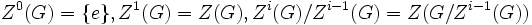# Upper central series is fastest ascending central series

## Statement

Suppose$G$ is a Nilpotent group (?) with a Central series (?) (written in ascending order as):$\{ e \} = H_0 \le H_1 \le H_2 \le \dots H_n = G$

Denote by$Z^i(G)$ the$i^{th}$ member of the Upper central series (?) of$G$, i.e.:$Z^0(G) = \{ e \}, Z^1(G) = Z(G), Z^i(G)/Z^{i-1}(G) = Z(G/Z^{i-1}(G))$

Then, we have:$Z^i(G) \ge H_i$

In particular, if$c$ is the Nilpotence class (?) of$G$, we have:$n \ge c$

## Proof

Given:$G$ is a Nilpotent group (?) with a Central series (?) (written in ascending order as):$\{ e \} \le H_0 \le H_1 \le H_2 \le \dots H_n = G$

Denote by$Z^i(G)$ the$i^{th}$ member of the Upper central series (?) of$G$, i.e.:$Z^0(G) = \{ e \}, Z^1(G) = Z(G), Z^i(G)/Z^{i-1}(G) = Z(G/Z^{i-1}(G))$

To prove:$Z^i(G) \ge H_i$

In particular, if$c$ is the Nilpotence class (?) of$G$, we have:$n \ge c$

Proof: We prove this by induction on$i$.

Base case for induction: For$i = 0$,$Z^0(G) = H_0 = \{ e \}$ so we're okay.

Induction step: Suppose$Z^i(G) \ge H_i$. We want to show that$Z^{i+1}(G) \ge H_{i+1}$.

Since$[G,H_{i+1}] \le H_i$, and$H_i \le Z^i(G)$, we see that under the projection$G \to G/Z^i(G)$, the image of elements of$H_i$ are in the center of$G/Z^i(G)$. Thus,$H_{i+1}Z^i(G)/Z^i(G)$ is in the center of$G/Z^i(G)$, so$H_{i+1}Z^i(G) \le Z^{i+1}(G)$ so$H_{i+1} \le Z^{i+1}(G)$ as required.

Since the nilpotence class$c$ is the smallest integer for which$Z^c(G) = G$,$H_n = G$ must force$n \ge c$.